# R语言中GLM(广义线性模型)，非线性和异方差可视化分析

• 链接函数（这实际上是在预测模型的关键）
• 分布或方差函数

``````­lin.mod = lm(dist~speed,data=cars)
``````

### 精算科学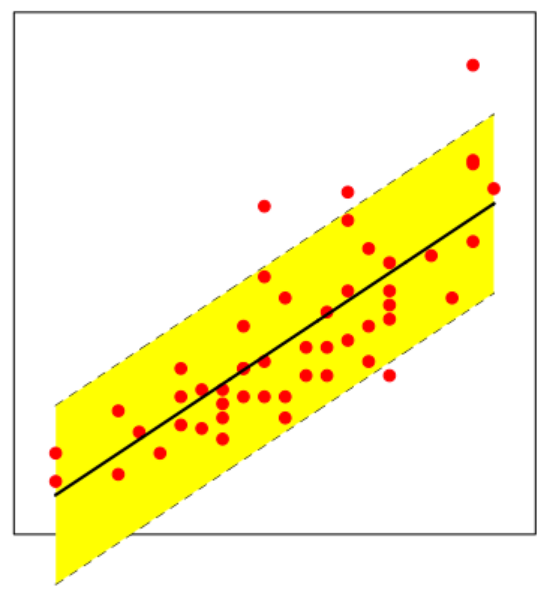​它将基于某些误差项生成与先前描述的模型相同的模型。该模型可以在下面看到，

``````C=trans3d(c(x,x),c(y,rev(y)),c(z,z0),mat)
polygon(C,border=NA,col="light blue",density=40)
C=trans3d(x,y,z0,mat)
lines(C,lty=2)
C=trans3d(x,y,z,mat)
lines(C,col="blue")}``````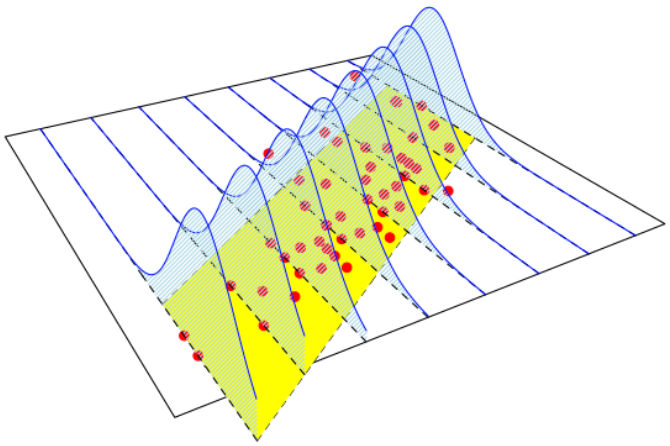``poisson.reg = glm(dist~speed,data=cars,family=poisson(link="log"))``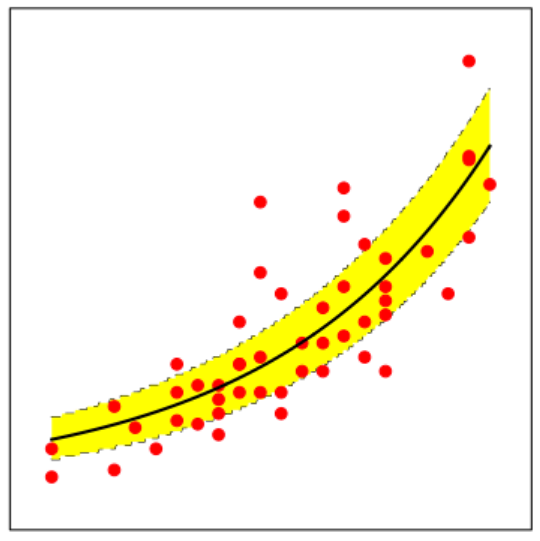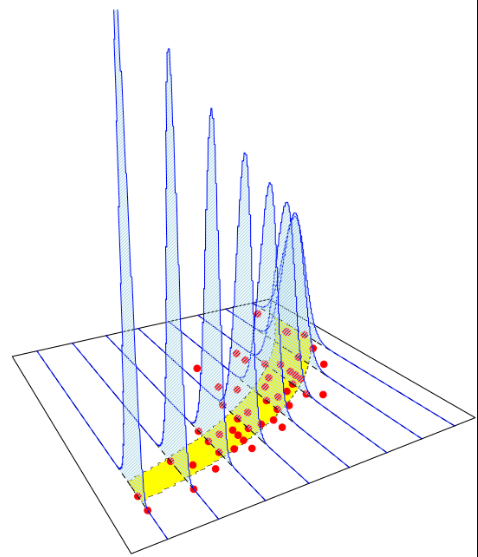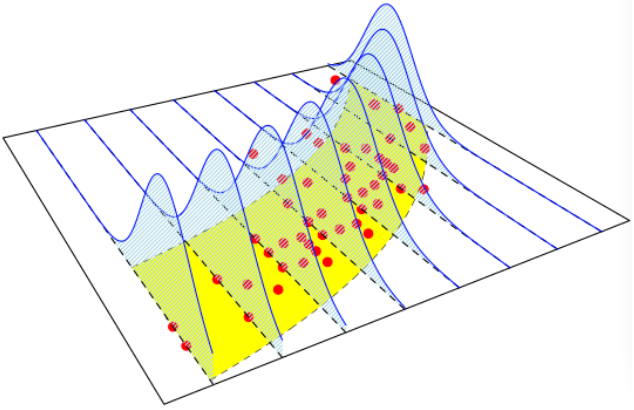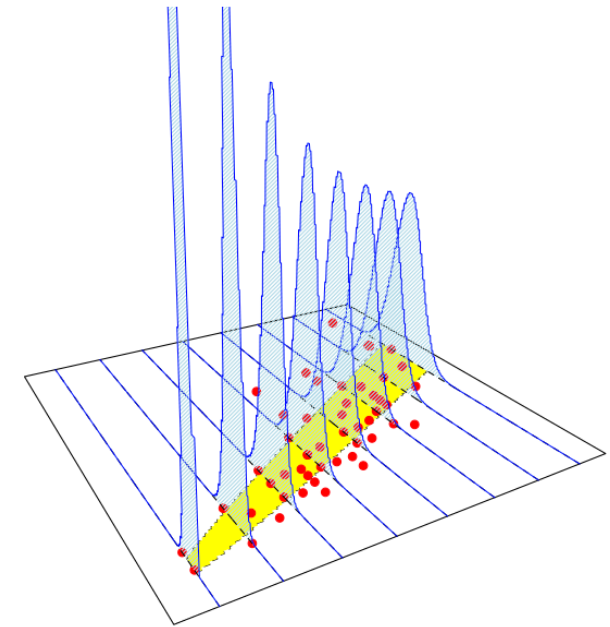Kaizong Ye拓端研究室（TRL）的研究员。

​非常感谢您阅读本文，如需帮助请联系我们！QQ在线咨询

15121130882

0571-63341498

## 关注有关新文章的微信公众号

This will close in 0 seconds